Example Questions

← Previous 1 3 4 5 6 7 8 9 23 24

Example Question #1 : Physics

A physicist performs a series of experiments to determine the relative magnitude of electric charge on four particles. A given particle is considered to have a higher magnitude of charge than another if it will push out (or draw in) a positive test charge farther than the other particle.

A particle that pushes the test charge has positive charge, while a particle that pulls (or draws in) the test charge has negative charge. This is known as the sign of the charge. Magnitude of charge is unrelated to sign.

The experiment is conducted on a horizontal axis that measures from 20m in total: from –10m on the left to +10m on the right, with a measurement of 0m in the middle.

Experiment 1

Particle A is placed at position –5m on the horizontal axis. The test charge has a specific magnitude of charge and is located at +3m on that same axis. The result of the experiment is that the test charge is displaced to +7.5m.

Experiment 2

Particle B is placed at position –8m on the horizontal axis. The test charge has the same magnitude of charge as the previous experiment and is located at 0m on that same axis. The result of the experiment is that the test charge is displaced to –7.5m.

Experiment 3

Particle C is placed at position 0m on the horizontal axis. The test charge has the same magnitude of charge as the previous experiment and is located at +8m on that same axis. The result of the experiment is that the test charge is displaced to +10m.

Experiment 4

Particle D is placed at position –5.5m on the horizontal axis. The test charge has the same magnitude of charge as the previous experiment and is located at +2.5m on that same axis. The result of the experiment is that the test charge is displaced to +7.5m.

It can be inferred from the experiment that __________.

All particles have an equal charge density.

Particle A and particle B have equal sign and magnitude.

Particle A and particle D have equal sign and magnitude.

All particles are placed an equal distance from the test charge.

All particles are placed an equal distance from the test charge.

Explanation:

Subtract the position of the particle from the initial position of the test charge.

In each experiment the result is 8m.

Example Question #1 : Physics

A physicist performs a series of experiments to determine the relative magnitude of electric charge on four particles. A given particle is considered to have a higher magnitude of charge than another if it will push out (or draw in) a positive test charge farther than the other particle.

A particle that pushes the test charge has positive charge, while a particle that pulls (or draws in) the test charge has negative charge. This is known as the sign of the charge. Magnitude of charge is unrelated to sign.

The experiment is conducted on a horizontal axis that measures from 20m in total: from –10m on the left to +10m on the right, with a measurement of 0m in the middle.

Experiment 1

Particle A is placed at position –5m on the horizontal axis. The test charge has a specific magnitude of charge and is located at +3m on that same axis. The result of the experiment is that the test charge is displaced to +7.5m.

Experiment 2

Particle B is placed at position –8m on the horizontal axis. The test charge has the same magnitude of charge as the previous experiment and is located at 0m on that same axis. The result of the experiment is that the test charge is displaced to –7.5m.

Experiment 3

Particle C is placed at position 0m on the horizontal axis. The test charge has the same magnitude of charge as the previous experiment and is located at +8m on that same axis. The result of the experiment is that the test charge is displaced to +10m.

Experiment 4

Particle D is placed at position –5.5m on the horizontal axis. The test charge has the same magnitude of charge as the previous experiment and is located at +2.5m on that same axis. The result of the experiment is that the test charge is displaced to +7.5m.

The results of Experiment 1 and 2 show that __________.

Particle A has the same magnitude of charge as particle B.

Particle A has a lower magnitude of charge than particle B.

Particle A has a higher magnitude of charge than particle B.

Particle A has a lower charge density than particle B.

Particle A has a lower magnitude of charge than particle B.

Explanation:

Particle A has a lower magnitude of charge than particle B because it displaced the test charge by a smaller amount.

In experiment 1, the test charge moved from +3m to +7.5m, a difference of 4.5m.

In experiment 2, the test charge moved from 0m to –7.5m, a difference of 7.5m.

The experiments give no insight into charge density.

Example Question #3 : Physics

A physicist performs a series of experiments to determine the relative magnitude of electric charge on four particles. A given particle is considered to have a higher magnitude of charge than another if it will push out (or draw in) a positive test charge farther than the other particle.

A particle that pushes the test charge has positive charge, while a particle that pulls (or draws in) the test charge has negative charge. This is known as the sign of the charge. Magnitude of charge is unrelated to sign.

The experiment is conducted on a horizontal axis that measures from 20m in total: from –10m on the left to +10m on the right, with a measurement of 0m in the middle.

Experiment 1

Particle A is placed at position –5m on the horizontal axis. The test charge has a specific magnitude of charge and is located at +3m on that same axis. The result of the experiment is that the test charge is displaced to +7.5m.

Experiment 2

Particle B is placed at position –8m on the horizontal axis. The test charge has the same magnitude of charge as the previous experiment and is located at 0m on that same axis. The result of the experiment is that the test charge is displaced to –7.5m.

Experiment 3

Particle C is placed at position 0m on the horizontal axis. The test charge has the same magnitude of charge as the previous experiment and is located at +8m on that same axis. The result of the experiment is that the test charge is displaced to +10m.

Experiment 4

Particle D is placed at position –5.5m on the horizontal axis. The test charge has the same magnitude of charge as the previous experiment and is located at +2.5m on that same axis. The result of the experiment is that the test charge is displaced to +7.5m.

If particle C and particle D are placed on the axis at the same time, accoring to the results of the experiment, what is likely to occur?

There would be no reaction.

The combined effect of their relative charge would displace a test charge located at 0m to +7m.

They would push (repel) each other.

They would draw in (attract) each other.

They would push (repel) each other.

Explanation:

The best answer is that they would push (repel) each other. We know from the effects of a positive particle on a positive test charge that like signs repel and unlike signs draw in (attract) each other.

We know the two particles have the same sign (possitive) because of how they affect the test charge in the experiments.

We cannot speculate about how the combined effect of their relative charges would displace a test charge without knowing their exact locations on the axis.

We know that there must be some reaction.

Example Question #1 : How To Find Research Summary In Physics

A physicist performs a series of experiments to determine the relative magnitude of electric charge on four particles. A given particle is considered to have a higher magnitude of charge than another if it will push out (or draw in) a positive test charge farther than the other particle.

A particle that pushes the test charge has positive charge, while a particle that pulls (or draws in) the test charge has negative charge. This is known as the sign of the charge. Magnitude of charge is unrelated to sign.

The experiment is conducted on a horizontal axis that measures from 20m in total: from –10m on the left to +10m on the right, with a measurement of 0m in the middle.

Experiment 1

Particle A is placed at position –5m on the horizontal axis. The test charge has a specific magnitude of charge and is located at +3m on that same axis. The result of the experiment is that the test charge is displaced to +7.5m.

Experiment 2

Particle B is placed at position –8m on the horizontal axis. The test charge has the same magnitude of charge as the previous experiment and is located at 0m on that same axis. The result of the experiment is that the test charge is displaced to –7.5m.

Experiment 3

Particle C is placed at position 0m on the horizontal axis. The test charge has the same magnitude of charge as the previous experiment and is located at +8m on that same axis. The result of the experiment is that the test charge is displaced to +10m.

Experiment 4

Particle D is placed at position –5.5m on the horizontal axis. The test charge has the same magnitude of charge as the previous experiment and is located at +2.5m on that same axis. The result of the experiment is that the test charge is displaced to +7.5m.

The results of experiments 3 and 4 show that __________.

Particle D has a higher charge than particle C.

Particle D has the opposite charge sign that particle has.

Particle D has a lower charge than particle C.

Particle D has the same charge as particle C.

Particle D has a higher charge than particle C.

Explanation:

We know that particles D and C have the same sign, as they pushed the test charge in the same direction.

We know that D has the higher magnitude because it displaced the test charge from +2.5m to +7.5m, a difference of 5m, while C displaced the test charge from +8m to +10m, a difference of 2m.

Example Question #2 : How To Find Research Summary In Physics

A physicist performs a series of experiments to determine the relative magnitude of electric charge on four particles. A given particle is considered to have a higher magnitude of charge than another if it will push out (or draw in) a positive test charge farther than the other particle.

A particle that pushes the test charge has positive charge, while a particle that pulls (or draws in) the test charge has negative charge. This is known as the sign of the charge. Magnitude of charge is unrelated to sign.

The experiment is conducted on a horizontal axis that measures from 20m in total: from –10m on the left to +10m on the right, with a measurement of 0m in the middle.

Experiment 1

Particle A is placed at position –5m on the horizontal axis. The test charge has a specific magnitude of charge and is located at +3m on that same axis. The result of the experiment is that the test charge is displaced to +7.5m.

Experiment 2

Particle B is placed at position –8m on the horizontal axis. The test charge has the same magnitude of charge as the previous experiment and is located at 0m on that same axis. The result of the experiment is that the test charge is displaced to –7.5m.

Experiment 3

Particle C is placed at position 0m on the horizontal axis. The test charge has the same magnitude of charge as the previous experiment and is located at +8m on that same axis. The result of the experiment is that the test charge is displaced to +10m.

Experiment 4

Particle D is placed at position –5.5m on the horizontal axis. The test charge has the same magnitude of charge as the previous experiment and is located at +2.5m on that same axis. The result of the experiment is that the test charge is displaced to +7.5m.

Which of the following represents the order of charge of the four particles, from highest to lowest?

B, D, A, C

D, A, C, B

B, D, C, A

A, B, C, D

B, D, A, C

Explanation:

Particle B displaced the test charge from 0m to –7.5m, a distance of 7.5m

Particle D displaced the test charge from +2.5m to +7.5m, a distance of 5m

Particle A displaced the test charge from +3m to +7.5m, a distance of 4.5m

Particle C displaced the test charge from +8m to +10m, a distance of 2m

Example Question #6 : Physics

A physicist performs a series of experiments to determine the relative magnitude of electric charge on four particles. A given particle is considered to have a higher magnitude of charge than another if it will push out (or draw in) a positive test charge farther than the other particle.

A particle that pushes the test charge has positive charge, while a particle that pulls (or draws in) the test charge has negative charge. This is known as the sign of the charge. Magnitude of charge is unrelated to sign.

The experiment is conducted on a horizontal axis that measures from 20m in total: from –10m on the left to +10m on the right, with a measurement of 0m in the middle.

Experiment 1

Particle A is placed at position –5m on the horizontal axis. The test charge has a specific magnitude of charge and is located at +3m on that same axis. The result of the experiment is that the test charge is displaced to +7.5m.

Experiment 2

Particle B is placed at position –8m on the horizontal axis. The test charge has the same magnitude of charge as the previous experiment and is located at 0m on that same axis. The result of the experiment is that the test charge is displaced to –7.5m.

Experiment 3

Particle C is placed at position 0m on the horizontal axis. The test charge has the same magnitude of charge as the previous experiment and is located at +8m on that same axis. The result of the experiment is that the test charge is displaced to +10m.

Experiment 4

Particle D is placed at position –5.5m on the horizontal axis. The test charge has the same magnitude of charge as the previous experiment and is located at +2.5m on that same axis. The result of the experiment is that the test charge is displaced to +7.5m.

Which of the particles is negatively charged?

Particle B

Particle D

Particle A

Particle C

Particle B

Explanation:

All of the particles are initially placed to the left of the test charge. Particle B is the only one that "draws in" the test charge to the left, from 0m to –7.5m (we are told initially that the axis runs from –10m on the left to 10m on the right, with 0m in the middle).

Example Question #7 : Physics

The photoelectric effect is a phenomenon that has led to many important scientific discoveries. Light of a particular wavelength is shined onto a piece of metal, showering the metal with photons. Wavelength is inversely related to a photon's energy. That is, with a smaller wavelength, the photon has greater energy. The wavelength of the light is decreased until a detector next to the metal senses that electrons are being ejected from the metal. This sensor also tells us how many electrons are ejected per second, which we call electrical current. At this point, any additional decrease in wavelength does not affect the number of electrons ejected. This point is called the metal's work function. However, if we then begin to increase the intensity of the light being shone (meaning the amount of light as opposed to the light's wavelength), the number of electrons picked up by the sensor increases.

According to the information in the passage, what can we infer would happen if the intensity of the light were decreased immediately after reaching the work function?

The current would remain, but would decrease.

The current would remain unchanged.

The current would stop.

The current would remain and increase.

The current would remain, but would decrease.

Explanation:

The correct answer is that the current would remain, but it would decrease. At the end of the passage, we are told that intensity of the light affects the current in a direct relationship. That is, more intensity means greater current. Therefore, as we have seen that the presence of a current is related to the wavelength of the light used, we know that the current would remain. Furthermore, as we have decreased the intensity, we know that this current would simply decrease.

Example Question #8 : Physics

The photoelectric effect is a phenomenon that has led to many important scientific discoveries. Light of a particular wavelength is shined onto a piece of metal, showering the metal with photons. Wavelength is inversely related to a photon's energy. That is, with a smaller wavelength, the photon has greater energy. The wavelength of the light is decreased until a detector next to the metal senses that electrons are being ejected from the metal. This sensor also tells us how many electrons are ejected per second, which we call electrical current. At this point, any additional decrease in wavelength does not affect the number of electrons ejected. This point is called the metal's work function. However, if we then begin to increase the intensity of the light being shone (meaning the amount of light as opposed to the light's wavelength), the number of electrons picked up by the sensor increases.

Light intensity can best be described as which of the following?

The speed at which photons of a light source travel

The color of light used

The number of photons being emitted by a light source

The energy of photons of a light source

The force photons exert on a surface

The number of photons being emitted by a light source

Explanation:

The correct answer is the number of photons. In the passage, we are told that the intensity of a light is related to the amount of light used. As light can be quantified in terms of photons, we know that this means that more intensity implies more photons. Any answer related to energy (including wavelength, force, and color) are incorrect because energy does not directly affect number of photons emitted and the speed of light is always the same.

Example Question #9 : Physics

The photoelectric effect is a phenomenon that has led to many important scientific discoveries. Light of a particular wavelength is shined onto a piece of metal, showering the metal with photons. Wavelength is inversely related to a photon's energy. That is, with a smaller wavelength, the photon has greater energy. The wavelength of the light is decreased until a detector next to the metal senses that electrons are being ejected from the metal. This sensor also tells us how many electrons are ejected per second, which we call electrical current. At this point, any additional decrease in wavelength does not affect the number of electrons ejected. This point is called the metal's work function. However, if we then begin to increase the intensity of the light being shone (meaning the amount of light as opposed to the light's wavelength), the number of electrons picked up by the sensor increases.

Based on the information in the passage, the term "work function" can be most accurately described as which of the following?

The point at which the energy of the photons hitting the metal has hit a limit

The point at which the energy of the photons hitting the metal is barely enough to eject electrons from that metal

The point at which the wavelength of light used has reached a limit

The point at which the metal has run out of electrons which can be ejected

The point at which no more electrons can or will leave the metal

The point at which the energy of the photons hitting the metal is barely enough to eject electrons from that metal

Explanation:

The correct answer is that it is the point at which the energy of the photons is barely enough to eject electrons. We know this because, as the passage says, decreasing the wavelength (increasing the energy) of the photons does not eject electrons until exactly this point. This implies that this gradual increase in photon energy passed the threshold we are calling the "work function" and led to electrons being ejected.

Example Question #10 : Physics

A particle accelerator functions by exerting a magnetic field on charged particles which are shot into the accelerator. The magnetic field causes the charged particles to move around in a circle of radiusthat can be predicted by the following equation, where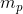is the mass of the particle in kilograms,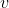is the initial speed at which the particle was shot in meters per second,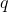is the charge of the particle in Coulombs, andis the strength of the magnetic field in Tesla.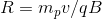If a given magnetic field's strength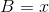and its radius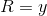, what would the radiusbe at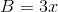?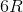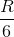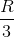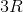The correct answer is. We know by the equation thatandare inversely related. As one increases, the other decreases, and vice versa. Therefore, ifis tripled,must be divided by.